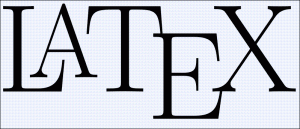# Top LaTeX MistakesIt is very easy to make mistakes when typesetting using LaTeX. John Cook and A.J. Hildebrand list some of my pet peeves. See below for my list.

1. Quotes:$"Hello"$ vs.$Hello"$
Notice that the quotation marks at the beginning of the first part are not going in the proper direction. To typeset quotes properly, you should type “Hello” instead of “Hello.” Notice that the ` is on the ~ key.
2. Parentheses, Brackets:$( dfrac{1}{2})$ vs.$left( dfrac{1}{2} right)$
Here, we have to use left( dfrac{1}{2} right) instead of just ( dfrac{1}{2} ). Using the left( and right) commands will cause TeX to automatically size the parentheses. This can also be used with

• left[ right]
• left{ right}
• left| right|
• leftlangle rightrangle
3. Inner Products:$$ vs.$langle p,q rangle$
Here, the second display (and, in my opinion, the proper display) can be obtained by  langle p,q rangle.
4. dx:$int f(x) dx$ vs.$int f(x) , dx$
Notice that the second one has a bit space between the f(x) and the dx. This can be obtained by int f(x) , dx.
5. Functions:$cos x$ vs.$cos x$
Notice that the first one does not have enough space between the cos and the x. We obtain the second one by cos x. Typically I will type cos(x) to obtain$cos(x)$. We can use this for the following functions as well:

• log x
• sin x
• tan x
6. Colon:$f : mathbb{R} to mathbb{R}$ vs.$f colon mathbb{R} to mathbb{R}$
Here, the : in the first one is offset a bit. You can obtain the second by typing  f colon mathbb{R} to mathbb{R}.

What LaTeX pet peeves do you have?

This entry was posted in Advice, Technology & Math. Bookmark the permalink.